## Plus Blog

December 22, 2013

It's that time of the year again when Santa needs to think about how to deliver all those presents to the people on Earth. Unfortunately his reindeers are a bit weak this year, having suffered some severe bouts of flu, and he knows he can't ask them to travel more than a million kilometres in total. Is there a route around the Earth that visits all those cities, towns and villages he needs to visit, but is less than a million kilometres long?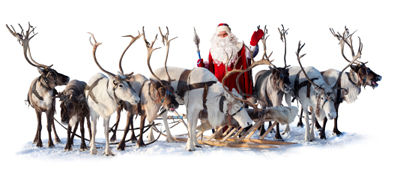A bit tired this year.

That, it turns out, is a very tricky question. One way of answering it would be to try out all different routes and work out their lengths. But since there are 7 billion people on Earth and he needs to visit every single dwelling that's totally unfeasible. It would take him until long after the Universe has ended to try all possible routes. Is there a clever algorithm Santa could use instead?

Obviously, the time it will take any algorithm to answer the question will depend on the number of places that need visiting — the more places, the longer it takes. So the question is whether there's an algorithm for which the time taken grows in a manageable, non-explosive way as the number of places grows — it's what complexity theorists call a polynomial time algorithm. If Santa were to ask such a complexity theorist he would find out that the class of all problems that can be solved in polynomial time has a name: it's called P.

Now Santa doesn't know if his problem of finding a route belongs to the class P. What's pretty obvious, though, is that if someone gave him a potential solution — a route they claim visits every place but is less than a million kilometres long — it would be easy to check whether that's true: he'd simply have to add up all the individual distances between places. A computer could do that quite easily. This means that Santa's problem belongs to a class of problems called NP. It consists of those problems for which potential answers can be checked in polynomial time, even though we might not know how to solve them from scratch in polynomial time.

But now suppose that Santa has a brainwave. Sitting down with a computer he constructs a polynomial time algorithm that finds the kind of route he is after. He would then have shown that his problem is not only a member of the NP class, but also of the P class. Amazingly, it would also hand Santa a polynomial time algorithm for all other problems in the NP class — because it turns out that his problem is a version of the famous travelling salesman problem, and that all the other problems in the NP class can be reduced to it. What is more, his algorithm would win Santa \$1 million: the question of whether the P class and the NP class of problems are equal is considered one of the hardest in mathematics, which is why the Clay Mathematics Institute as offered \$1 million to anyone who solves it.

Unfortunately Santa doesn't have the time to do any of this, and he's not a computer expert anyway. So he just takes an educated guess at what route to take and gets going, hoping for the best. It's a shame really because if he'd been able to show that P=NP he would have held the key to many of the world's secret communications, including everyone's bank details that are sent encrypted over the internet. This is because NP problems are used as the key for such encryptions, with the secrecy relying on the fact that the problems are very hard to crack. You can find out more in the Plus articles The travelling salesman and Safety in numbers.

December 21, 2013

Raging rivers and thundering waves are exciting and frightening and for mathematicians they are a massive problem. Suppose you've got a turbulent mountain stream — if you're a mathematician, you'll want to know if you can describe the flow of the water using a mathematical equation. Given a point in space (that is somewhere in the stream) and a point in time (say 5 minutes from now), you would like to know the velocity and maybe also the pressure of the water at that point in time and space.Image: misty.

Scientists believe that turbulence is described to a reasonable level of accuracy by a very famous set of equations, known as the Navier-Stokes equations. These are partial differential equations which relate changes in velocity, changes in pressure and the viscosity of the liquid. To find the velocity and pressure of your liquid, you have to solve them.

But that's no easy feat. Exact solutions to the equations — solutions that can be written down as mathematical formulae — exist only for simplified problems that are of little or no physical interest. For most practical purposes, approximate solutions are found through computer simulations — essentially through educated guess-work — that require immense computing power.

Plus there is an additional problem that makes numerical difficulties pale into insignificance: no one knows if exact mathematical solutions even exist in all cases. And if they do exist, we still don't know if they involve oddities, such as discontinuities or infinities, that don't square with our intuition of how a liquid should behave.

It's these difficulties that have turned the understanding of the Navier-Stokes equations into one of the seven Millennium Problems posed by the Clay Mathematics Institute. Whoever proves or disproves the existence of finite and smooth solutions is set to earn a million dollars.

All this might seem strange, even scary, given that the equations are used all over the place, all the time — meteorology and aircraft design are just two examples. The fact is that, in the cases we can compute, approximate solutions do seem to give an accurate description of the motion of fluids. What we don't know is what, if anything, the model given by the Navier-Stokes equations tells us about the exact nature of fluid flow.

You can find out more about turbulence on Plus:

December 20, 2013

The central idea of applied statistics is that you can say something about a whole population by looking at a smaller sample. Without this idea there wouldn't be opinion polls, the social sciences would be stuffed, and there would be no way of testing new medical drugs, or the safety of bridges, etc, etc. It's the central limit theorem that is to a large extent responsible for the fact that we can do all these things and get a grip on the uncertainties involved.

Suppose that you want to know the average weight of the population in the UK. You go out and measure the weight of, say, 100 people whom you've randomly chosen and work out the average for this group — call this the sample average. Now the sample average is supposed to give you a good idea of the nation's average. But what if you happened to pick only fat people for your sample, or only very skinny ones?

To get an idea of how representative your average is likely to be, you need to know something about how the average weight of 100-people-samples varies over the population: if you took lots and lots of samples of size 100 and worked out the average weight for each, then how variable would this set of numbers be? And what would its average (the average of averages) be compared to the true average weight in the population?

For example, suppose you know that if you took lots and lots of 100- people-samples and wrote down the average weight of each sample, you'd get all values from 10kg to 300kg in equal proportion. Then this would tell you that your method of estimating the overall average by taking one sample of a 100 people isn't a very good one, because there's too much variability — you're just as likely to get any of the possible values, and you don't know which one is closest to the true average weight in the population.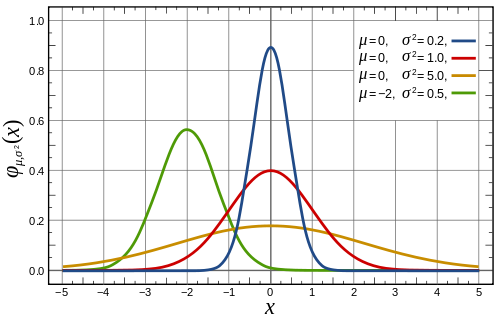Four versions of the normal distribution with different means and variances.

So how can we say anything about the distribution of 100-people-averages — called the sampling distribution — when we don't know anything about the distribution of weight across the population? This is where the central limit theorem comes in: it says that for a big enough sample (usually sampling 30 people is good enough) your sampling distribution is approximated by a normal distribution — this is the distribution with the famous bell shape.

The mean of this normal distribution (the average of averages corresponding to the tip of the bell) is the same as the mean in the population (the average weight of the population). The variance of this normal distribution, that is how much it varies about the mean (indicated by the width of the bell), depends on the sample size: the larger the sample, the smaller the variance. There's an equation which gives the exact relationship.

So if your sample size is big enough (100 would certainly do since it's bigger than 30), then the relatively small variance of the normal sampling distribution means that the average weight you observe is close to the mean of that normal distribution (since the bell is quite narrow). And since the mean of that normal distribution is equal to the true average weight across the population, your observed average is a good approximation of the true average.

You can make all this precise, for example you can say exactly how confident you are that the true average is within a certain distance of your sample average, and you can also use the result to calculate how large a sample you need to get an estimate of a given accuracy. It's the central limit theorem that lends precision to the art of statistical inference, and it's also behind the fact that the normal distribution is so ubiquitous.

The central limit theorem is actually a bit more general than we've let on here. See here for a precise statement.

December 19, 2013

The dramatic curved surfaces of some of the iconic buildings created in the last decade, such as 30 St Mary's Axe (AKA the Gherkin) in London, are only logistically and economically possible thanks to mathematics. Curved panels of glass or other material are expensive to manufacture and to fit. Surprisingly, the curved surface of the Gherkin has been created almost entirely out of flat panels of glass — the only curved piece is the cap on the very top of the building. And simple geometry is all that is required to understand how.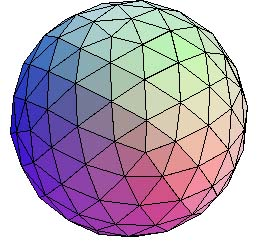A geodesic sphere.

One way of approximating a curved surface using flat panels is using the concept of geodesic domes and surfaces. A geodesic is just a line between two points that follows the shortest possible distance — on the Earth the geodesic lines are great circles, such as the lines of longitude or the routes aircraft use for long distances. A geodesic dome is created from a lattice of geodesics that intersect to cover the curved surface with triangles. The mathematician Buckminster Fuller perfected the mathematical ideas behind geodesic domes and hoped that their properties — greater strength and space for minimum weight — might be the future of housing.

To try to build a sphere out of flat panels, such as a geodesic sphere, you first need to imagine an icosahedron (a polyhedron made up of 20 faces that are equilateral triangles) sitting just inside your sphere, so that the points of the icosahedron just touch the sphere's surface. An icosahedron, with its relatively large flat sides, isn't going to fool anyone into thinking it's curved. You need to use smaller flat panels and a lot more of them.

Divide each edge of the icosahedron in half, and join the points, dividing each of the icosahedron's faces into four smaller triangles. Projecting the vertices of these triangles onto the sphere (pushing them out a little til they two just touch the sphere's surface) now gives you a polyhedron with 80 triangular faces (which are no longer equilateral triangles) that gives a much more convincing approximation of the curved surface of the sphere. You can carry on in this way, dividing the edges in half and creating more triangular faces, until the surface made up of flat triangles is as close to a curved surface as you would like.

Find out more about the Gherkin and other iconic buildings on Plus.

December 18, 2013

How would you go about adding up all the integers from 1 to 100? Tap them into a calculator? Write a little computer code? Or look up the general formula for summing integers?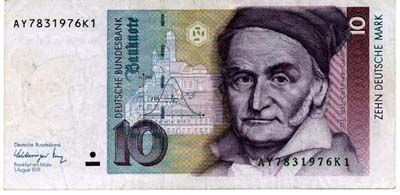Carl Friedrich Gauss as depicted on the (now defunct) German 10 Marks note.

Legend has it that the task of summing those numbers was given to the young Carl Friedrich Gauss by his teacher at primary school, as a punishment for misbehaving. Gauss didn't have a calculator or computer, no one did at that time, but he came up with the correct answer within seconds. Here's how he did it.

Notice that you can sum the numbers in pairs, starting at either end. First you add 1 and 100 to get 101. Next it's 2 and 99, giving 101 again. The same for 3 and 98. Continuing like this, the last pair you get is 50 and 51 and they give 101 again. Altogether there are 50 pairs all adding to 101, so the answer is 50 x 101 = 5050. Easy — if you're Gauss.

December 17, 2013

Sequences of numbers can have limits. For example, the sequence 1, 1/2, 1/3, 1/4, ... has the limit 0 and the sequence 0, 1/2, 2/3, 3/4, 4/5, ... has the limit 1.

But not all number sequences behave so nicely. For example, the sequence 1/2, 1/3, 2/3, 1/4, 3/4, 1/5, 4/5, ... keeps jumping up and down, rather than getting closer and closer to one particular number. We can, however, discern some sort of limiting behaviour as we move along the sequence: the numbers never become larger than 1 or smaller than 0. And what's more, moving far enough along the sequence, you can find numbers that get as close as you like to both 1 and 0. So both 0 and 1 have some right to be considered limits of the sequence — and indeed they are: 1 is the limit superior and 0 is the limit inferior, so-called for obvious reasons.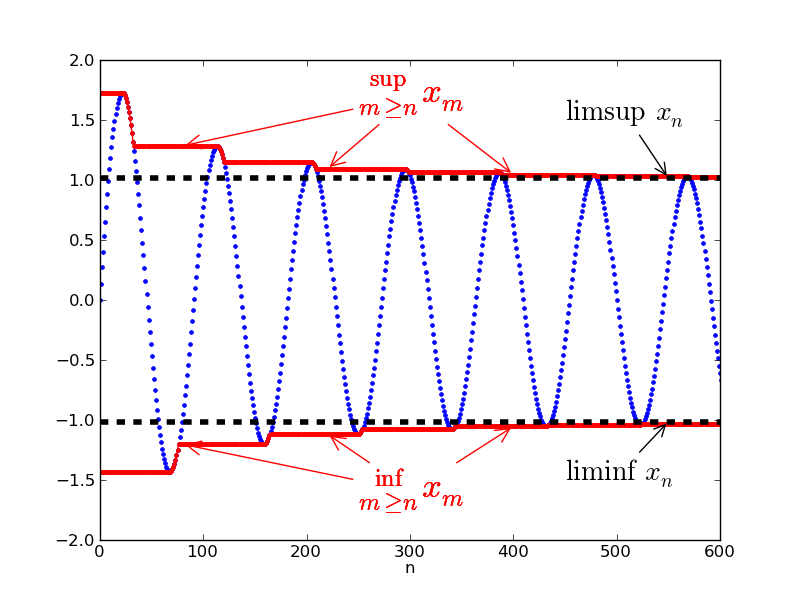But can you define these limits superior and inferior for a general sequence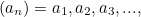for example the one shown in the picture? Here’s how to do it for the limit superior. First look at the whole sequence and find its least upper bound: that’s the smallest number that’s bigger than all the numbers in the sequence. Then chop off the first number in the sequence,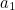, and again find the least upper bound for the new sequence. This might be smaller than the previous least upper bound (if that was equal to), but not bigger. Then chop off the first two numbers and again find the least upper bound.

Keep going, chopping off the first three, four, five, etc numbers, to get a sequence of least upper bounds (indicated by the red curve in the picture). In this sequence every number is either equal to or smaller than the number before. The limit superior is defined to be the limit of these least upper bounds. It always exists: since the sequence of least upper bounds is either constant or decreasing, it will either approach minus infinity or some other finite limit. The limit superior could also be equal to plus infinity, if there are numbers in the sequence that get arbitrarily large.

The limit inferior is defined in a similar way, only that you look at the sequence of greatest lower bounds and then take the limit of that.

You can read more about the limits inferior and superior in the Plus article The Abel Prize 2012.